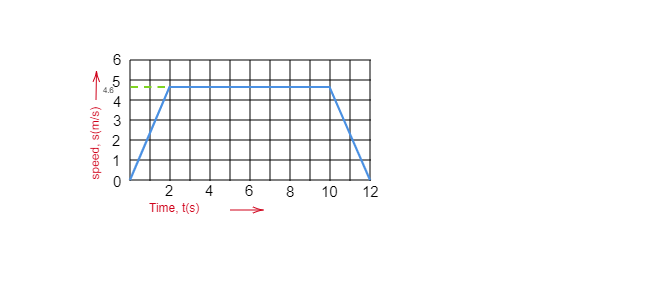(i) during the first two seconds?
(ii) between second and tenth second?
(iii) during the last two seconds?"
">

# The speed-time graph of an ascending passenger lift is given alongside. What is the acceleration of the lift:(i) during the first two seconds?(ii) between second and tenth second?(iii) during the last two seconds?"

(i). Acceleration during the first two seconds $=\frac{4.6-0}{2-0}=\frac{4.6}{2}=2.3\ m/s^2$

(ii). Acceleration during second and tenth seconds $=\frac{4.6-4.6}{10-2}=0$

(iii). Acceleration during last 2 seconds $=\frac{0-4.6}{12-10}$

$=\frac{-4.6}{2}$

$=-2.3\ m/s^2$   [-ve sign indicates retardation]

Updated on: 10-Oct-2022

27 Views